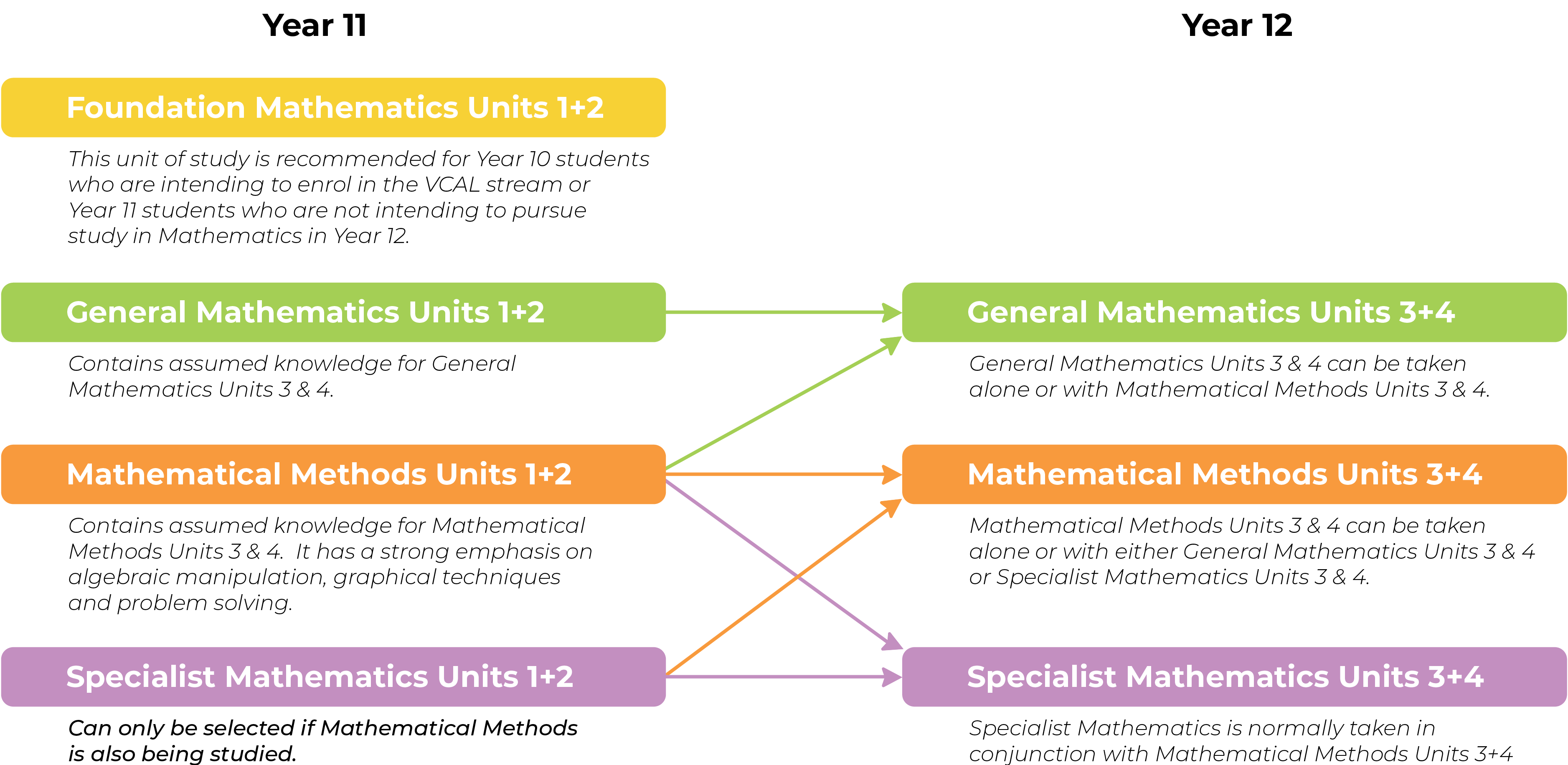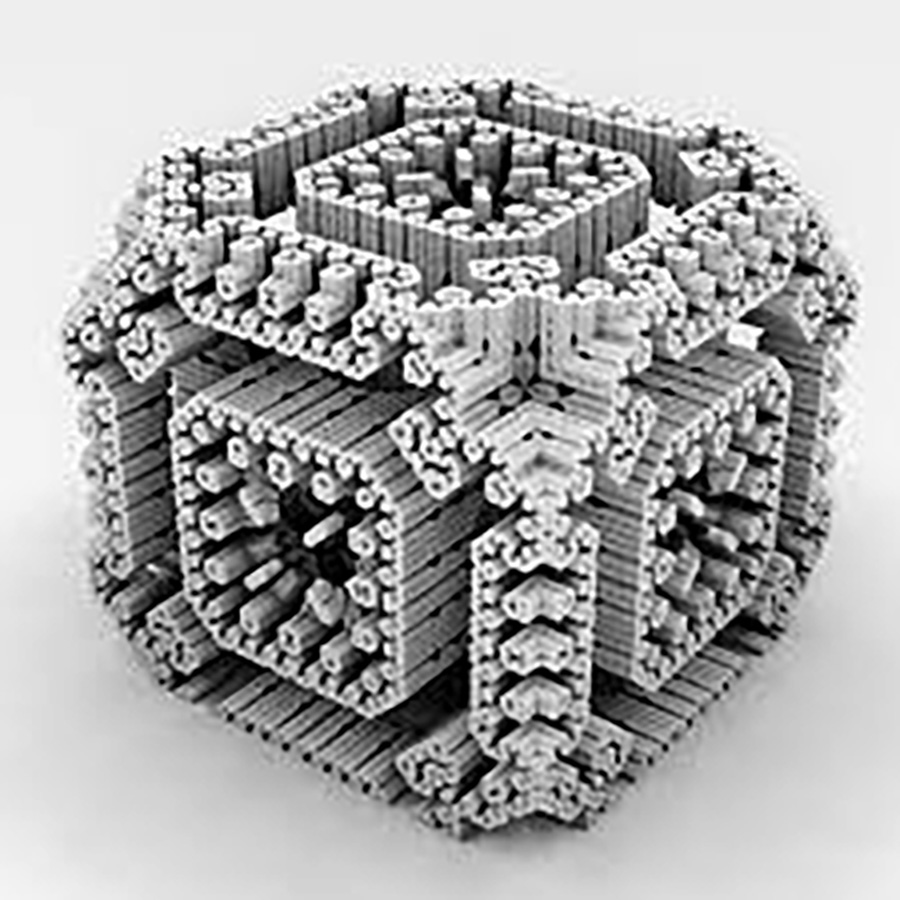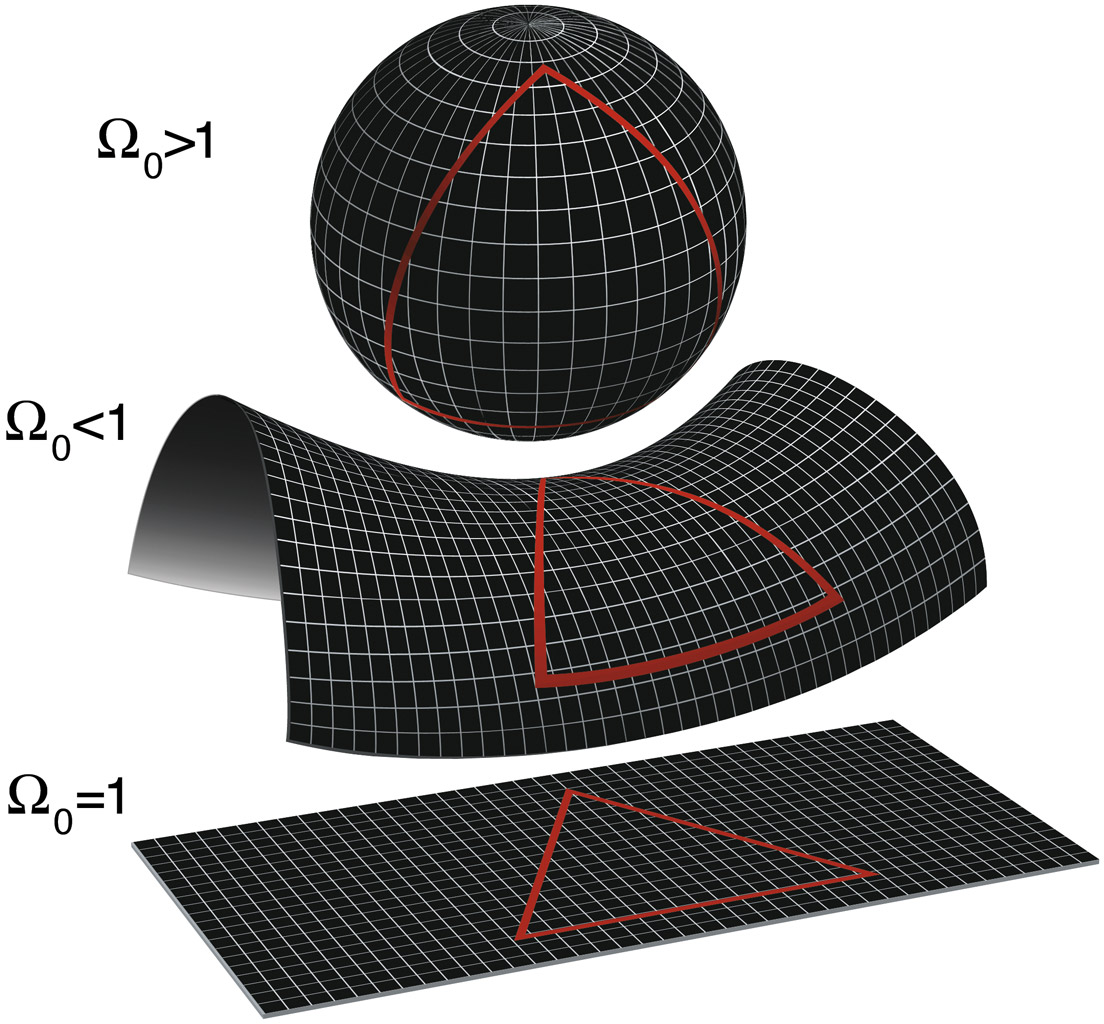# Mathematics Pathways# Foundation Mathematics#### Overview

Foundation Mathematics focuses on providing students with the ability to manage and solve problems encountered in practical contexts in everyday life at home, in the community, at work and in study.

It is recommended for Year 10 students who are intending to enrol in the VCAL stream in Year 11 or a Year 11 student who is not enrolled in General Mathematics Units 1 & 2 or Mathematical Methods Units 1 & 2.

This course by itself is not an adequate preparation for Units 3 & 4 General Mathematics, Mathematical Methods or Specialist Mathematics.

#### Areas of Study

The areas for study in both units are

• Algebra, Number & Structure
• Data Analysis, Probability & Statistics
• Discrete Mathematics
• Space & Measurement

#### Assessment

Assessment tasks will be drawn from:

• portfolio
• assignments
• tests (with and without technology)
• solutions to sets of worked questions
• summary or review notes
• mathematical investigations

# General Mathematics Unit 1+2#### Overview

General Mathematics Units 1 and 2 cater for a range of student interests and provides preparation for the study of VCE General Mathematics Units 3 & 4.

On completion of these units the student should be able to:

• Define and explain key concepts as specified in the content from the areas of study and use this knowledge to solve routine application problems.
• Select and apply mathematical facts, concepts, models and techniques from the topics covered in the unit to investigate, analyse and model situations in a range of non-routine contexts.
• Use a CAS calculator to develop mathematical ideas, produce results and carry out analysis in situations requiring problem-solving, modelling or investigative techniques or approaches

#### Unit Prerequisites

Students are expected to have completed Year 10 mathematics.

#### Areas of Study

There are four areas of study:

• Data analysis, probability and statistics
• Algebra, number and structure
• Functions, relations and graphs
• Discrete mathematics

#### Unit Assessment

Assessment tasks will be drawn from:

• assignments
• tests
• solutions to sets of worked questions
• summary or review notes
• mathematical investigations

#### Pathways

General Mathematics Units 1 & 2 allows students to study General Mathematics Units 3 & 4. A study of Mathematics is often a pre-requisite or an advantage to study beyond secondary school.

# Mathematical Methods Unit 1+2#### Mathematical Methods Units 1 & 2

Mathematical Methods provide background for further study in areas such as: science, technology, engineering and mathematics (STEM), humanities, economics and medicine.  It is a pre-requisite for study of Mathematical Methods Units 3 & 4.

#### Overview

On completion of these units the student should be able to:

• Define and explain key concepts as specified in the areas of study and apply a range of related mathematical routines and procedures
• Apply mathematical processes in non-routine contexts and analyse and discuss these applications of mathematics
• Use a CAS calculator to produce results and carry out analysis in situations requiring problem solving, modelling or investigative techniques or approaches.

#### Unit Prerequisites

All students must have completed Year 10 Mathematics to a satisfactory standard. It is strongly advised that they have completed their studies in Year 10 Maths A. Mathematical Methods is a challenging subject and a strong ability and / or determination are vital to taking on this challenge.

#### Areas of Study

• Functions, relations and graphs
• Algebra, number and structure
• Calculus
• Data analysis, probability and statistics

#### Unit Assessment

Assessment tasks will be drawn from:

• assignments
• tests
• solutions to sets of worked questions
• summary or review notes
• mathematical investigations

#### Pathways

Mathematical Methods Units 1 & 2 allows students to study Mathematical Methods Units 3 & 4 and General Mathematics Units 3 & 4. Other subjects that complement Mathematical Methods are: Specialist Mathematics, Chemistry, Physics, Algorithmics.

# Specialist Mathematics Unit 1+2#### Specialist Mathematics Units 1 & 2

Specialist Mathematics Units 1 & 2 is a course of study for students who wish to undertake an in-depth study of mathematics, with an emphasis on concepts, skills and processes related to mathematical structure, modelling, problem-solving, reasoning and proof.  It is a pre-requisite for study of Specialist Mathematics Units 3 & 4.

#### Overview

On completion of these units the student should be able to:

• Define and explain key concepts as specified in the areas of study and apply a range of related mathematical routines and procedures
• Apply mathematical processes in non-routine contexts and analyse and discuss these applications of mathematics
• Use a CAS calculator to produce results and carry out analysis in situations requiring problem solving, modelling or investigative techniques or approaches

#### Unit Prerequisites

Specialist Mathematics may only be taken in conjunction with Mathematical Methods Units 1 & 2.

It is strongly advised that students who are thinking of choosing Specialist Mathematics Units 3 & 4 complete the sequence of Specialist Units 1 & 2.

#### Areas of Study

• Algebra, structure and number
• Discrete mathematics
• Data analysis, probability and statistics
• Space and measurement
• Functions, relations and graphs

#### Unit Assessment

Assessment tasks will be drawn from

• assignments
• tests
• solutions to sets of worked questions
• summary or review notes
• mathematical investigations

#### Pathways

Specialist Mathematics Units 1 & 2 provides a comprehensive background for Mathematical Methods Units 3 & 4 and Specialist Mathematics Units 3 & 4. These subjects are strongly recommended for students hoping to enter the areas of Science Engineering, Applied Mathematics or Statistics.

# General Mathematics Unit 3+4#### General Mathematics Units 3 & 4

This area of study was previously known as Further Mathematics Units 3 & 4.
General Mathematics Units 3 and 4 focus on real-life application of mathematics.

#### Overview

On completion of these units the student should be able to:

• Define and explain key concepts as specified in the areas of study and use this knowledge to apply a range of related mathematical techniques and models in routine contexts
• Select and apply mathematical concepts, models and techniques in a range of contexts of increasing complexity
• Use a CAS calculator to produce results and carry out analysis in situations requiring problem-solving, modelling or investigative techniques or approaches.

#### Unit Prerequisites

This subject may be selected by students who have undertaken either General Mathematics 1 & 2 and or Mathematical Methods 1 & 2.

Highly able students who successfully undertake Mathematics A in Year 10 may apply to accelerate into General Mathematics Units 3 & 4 in Year 11. If acceleration is approved, the student must also select Maths Methods Units 1 & 2.

#### Areas of Study

All areas of study in General Mathematics are prescribed and compulsory.

• Data analysis
• Recursion and financial modelling
• Matrices
• Graphs and networks

Unit Assessment

School assessed coursework contributes 40% to the study score

• Unit 3 consists of an Application task of 4–6 hours’ duration over a period of 1–2 week and a problem solving or modelling task of 2–3 hours’ duration over a period of 1 week.
• Unit 4 consists of two problem solving or modelling tasks of 2–3 hours’ duration over a period of 1 week.

Units 3 and 4 are also assessed by two end of year examinations which contribute 60% to the study score.

• Exam 1 will contribute 30% to the study score.
• Exam 2 will contribute 30% to the study score.

# Mathematical Methods Unit 3+4#### Mathematical Methods Units 3 & 4

Mathematical Methods provide background for further study in areas such as: science, technology, engineering and mathematics (STEM), humanities, economics and medicine.

#### Overview

On completion of these units the student should be able to:

• Define and explain key concepts as specified in the areas of study and use this knowledge to apply a range of related mathematical techniques and models in routine contexts
• Select and apply mathematical concepts, models and techniques in a range of contexts of increasing complexity
• Use a CAS calculator to produce results and carry out analysis in situations requiring problem-solving, modelling or investigative techniques or approaches.

#### Unit Prerequisites

Students undertaking Unit 3 and 4 of Mathematical Methods are expected to have confidence in their abilities and a strong understanding of Unit 1 & 2 Mathematical Methods.

#### Areas of Study

• Functions, relations and graphs
• Algebra, number and structure
• Calculus
• Data analysis, probability and statistics

#### Assessment

School assessed coursework contributes 40% to the study score.

• Unit 3 consists of an application task of 4–6 hours’ duration over a period of 1–2 weeks.
• Unit 4 consists of two problem solving or modelling tasks of 2–3 hours’ duration over a period of 1 week.

Units 3 and 4 are also assessed by two end of year examinations which contribute 60% to the study score.

• Exam 1 will contribute 20% to the study score
• Exam 2 will contribute 40% to the study score

#### Pathways

Other subjects that are often seen to complement Mathematical Methods are: Specialist Mathematics, Physics, Chemistry.

# Specialist Mathematics Unit 3+4#### Specialist Mathematics Units 3 & 4

Specialist Mathematics provides for the study of various mathematical structures, reasoning and proof. The areas of study in Units 3 and 4 extend content from Mathematical Methods Units 3 and 4.

#### Overview

On completion of these units the student should be able to:

• Define and explain key concepts as specified in the areas of study and apply a range of related mathematical routines and procedures
• Apply mathematical processes in non-routine contexts and analyse and discuss these applications of mathematics
• Use a CAS calculator to produce results and carry out analysis in situations requiring problem solving, modelling or investigative techniques or approaches

#### Unit Prerequisites

Study of Specialist Mathematics Units 3 and 4 requires concurrent study or previous completion of Mathematical Methods Units 3 and 4.

It is strongly advised that students who are thinking of choosing Specialist Mathematics Units 3 & 4 complete the sequence of Specialist Units 1 & 2.

#### Areas of Study

• Discrete mathematics
• Functions, relations and graphs
• Algebra, number and structure
• Calculus
• Space and measurement
• Data analysis, probability and statistics

#### Assessment

School assessed coursework contributes 40% to the study score.

• Unit 3 consists of an application task of 4–6 hours’ duration over a period of 1–2 weeks.
• Unit 4 consists of two problem solving or modelling tasks of 2–3 hours’ duration over a period of 1 week.

Units 3 and 4 are also assessed by two end of year examinations which contribute 60% to the study score.

• Exam 1 will contribute 20% to the study score
• Exam 2 will contribute 40% to the study score

#### Pathways

Other subjects that are often seen to complement Specialist Mathematics are: Mathematical Methods, Physics, Chemistry.